×

Search anything:

# Fuzzy Relations, Propositions, Implications and Inferences

#### data science Machine Learning (ML)FREE BOOK -> Problems for the day before your Coding Interview (on Amazon)

In this article, we will first learn about fuzzy relations and the different types of operations that can be performed on them. Then, we will learn about the truth values of fuzzy propositions, about fuzzy implications or if-then rules, and finally, we will learn how to draw meaningful inferences from fuzzy systems.

## Fuzzy Relations

A fuzzy relation is defined as the cartesian product of fuzzy sets. If we have two fuzzy sets, on different universes of discourse, say:
A, on universe of discourse X, with μA(x) | x ∈ X
B, on universe of discourse Y, with μB(y) | y ∈ Y
Then, R = (A × B) ⊂ (X × Y), where the membership function of R is given by:
μR(x,y) = µA×B(x,y) = min {µA(x),µB(y)}

In other words, the degree of membership of each element of the newly obtained relation is the minimum degree of membership of its corresponding elements in the two fuzzy sets. To understand this better, let us take a look at an example.

If we have:
A = {(a1, 0.2), (a2, 0.7), (a3, 0.4)}
B = {(b1, 0.5), (b2, 0.6)}
Then, the relation R (AxB) would be: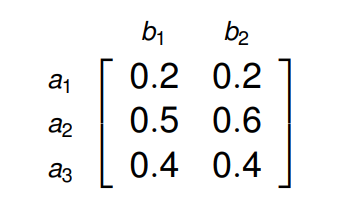When comparing a1 in set A and b1 in set B, we find that 0.2 is a smaller value than 0.5, which is why in the relation R, the degree of membership of the element corresponding to a1 and b1 is equal to 0.2. Similarly, we can find the degrees of membership of the rest of the elements.

## Operations on Fuzzy Relations

Let us assume that R and S are two fuzzy relations on A x B. Listed below are a few operations that can be performed on R and S.

### Union

µR∪S(a,b) = max {µR(a,b), µS(a,b)}

The union operation can only be performed if the relational matrices R and S are of the same order. Here, the degree of membership of each element of R∪S is the maximum degree of membership of its corresponding elements in R and S.

### Intersection

µR∩S(a,b) = min {µR(a,b), µS(a,b)}

The intersection operation can also only be performed in the relational matrices R and S are of the same order. Here, the degree of membership of each element of R∩S is the minimum degree of membership of its corresponding elements in R and S. Thus, we can say that R∩S is analogous to RxS.

### Complement

µR'(a,b) = 1 − µR(a,b)

The complement operation is performed on a singular relation. Here, the degree of membership of each element of the relation (after we perform the complement operation) is equal to one minus the previous degree of membership.

### Composition

T = R◦S, where:
µ(R◦S) = max y∈Y { min (µR(x,y), µS(y,z)) }

The composition operation is the most important operation that we will learn about in this article, as it is used extensively in drawing inferences from fuzzy systems. It is also called the max-min operation. Here, if R is defined on X x Y and S is defined on Y x Z, then the degree of membership of each element in R◦S (X x Z) is obtained by first finding the minimum degree of membership corresponding to the elements of the two relational matrices R and S (for all y∈Y, the common term in the two relational matrices), and then finding the maximum degree of membership. This will be more clear when we take a look at an example.

If we have:
X = (x1, x2, x3)
Y = (y1, y2)
Z = (z1, z2, z3)
And the two relations R and S are given as: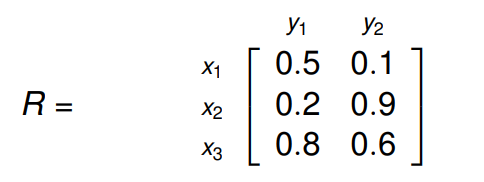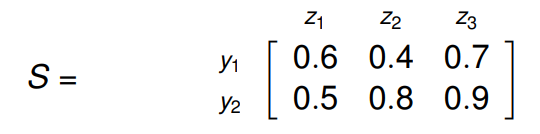Then, R◦S will be: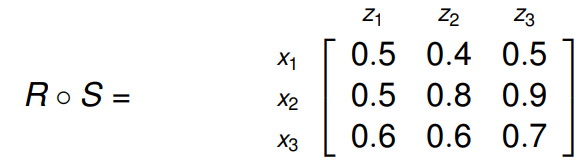To get the first element of R◦S, at index (0,0):
We first find the minimum of (0.5, 0.6) and (0.1, 0.5). Thus, we get (0.5) and (0.1). Now, we find the maximum out of these, which is (0.5). Similarly, we can find the degrees of membership of the rest of the elements.

## Fuzzy Propositions

Now that we have a clear idea on how to perform basic operations on fuzzy relations, we will talk about fuzzy propositions. A fuzzy proposition, much like a classical proposition is a statement that has a truth value. The difference between classical propositions and fuzzy propositions is that classical propositions can have a truth value of either 0 or 1, that is, they can either be entirely false or entirely true. Fuzzy propositions, on the other hand, can have truth values in the range [0, 1].

For example, if we we have a proposition P, defined as:
P - The weather is pleasant.
T(P) = 0, if absolutely false.
T(P) = 0.2, if mostly false.
T(P) = 0.4, if partially false.
T(P) = 0.6, if partially true.
T(P) = 0.8, if mostly true.
T(P) = 1, if absolutely true.
Where T(P) represents the truth value of the proposition.

Traditionally, most of us have extensively dealt with two-valued logic. For example:
0 0
0 1
1 0
1 1

However, when we deal with fuzzy propositions, we will see ourselves often dealing with multi-valued logic. For example, if we extend our logic to include 0, 1/2 and 1, we will have:
0 0
0 1/2
1/2 0
1/2 1/2
0 1
1 0
1 1
1/2 1
1 1/2

Listed below are a few operations that can be performed on propositions: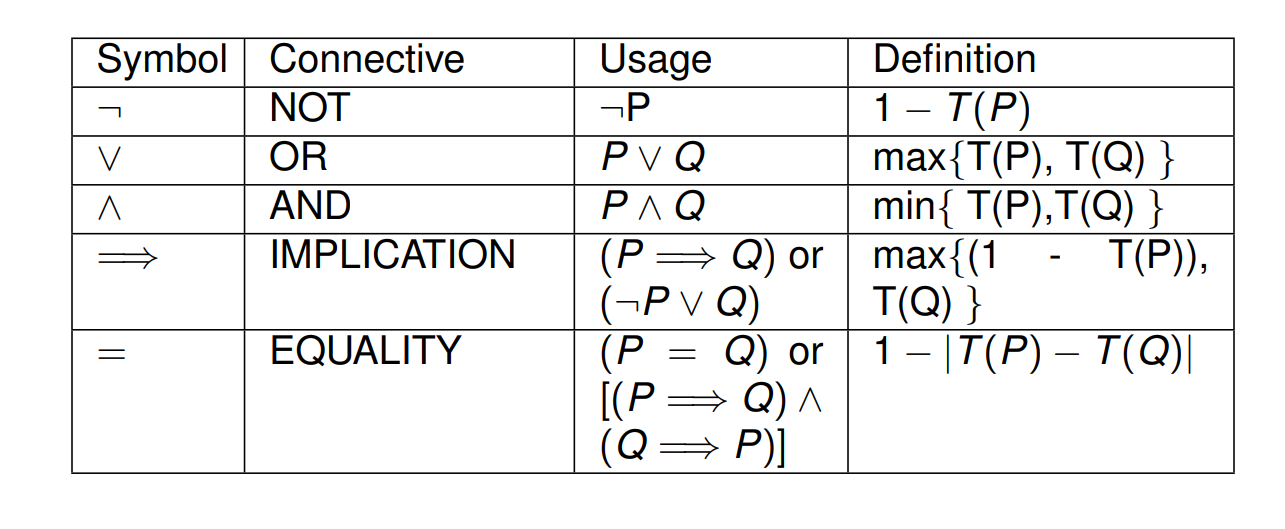If we perform these operations on three-valued logic, we will get the following results: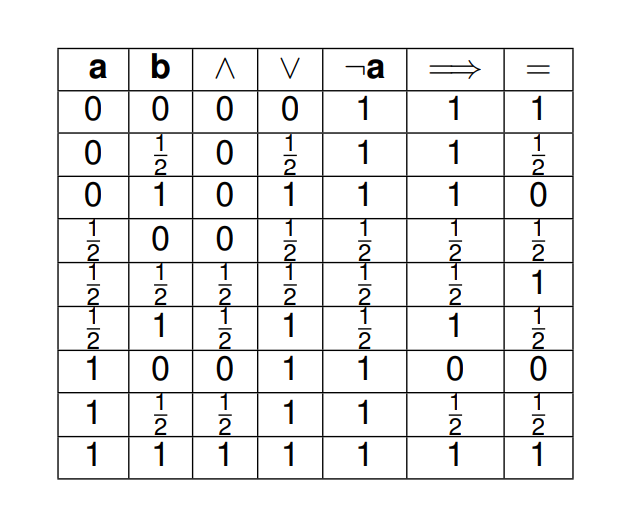The operations used in the above table are:
AND (∧), OR (∨), NOT (¬), IMPLICATION (⇒) and EQUAL TO (=).

Let us take a look at these operations being used on actual propositions. If we have:
P - Max is fast.
T(P) = 0.8
Q - Daniel is fast.
T(Q) = 0.6

Then, to find the truth value of the following statements, we have to use the above operators:

1. 'Max is not fast.'
T(¬P) = 1 − T(P) = 0.2

2. 'Max is fast and so is Daniel.'
T(P ∧ Q) = min{T(P),T(Q)} = 0.6

3. 'Either Max is fast or Daniel is.'
T(P ∨ Q) = max{T(P),T(Q)} = 0.8

4. 'If Max is fast, then so is Daniel.'
T(P ⇒ Q) = max{1−T(P),T(Q)} = 0.6

## Fuzzy Implications

A fuzzy implication, also known as a fuzzy if-then rule or a fuzzy conditional statement, takes the form:
If x is A then y is B.
Here, A and B are linguistic variables (defined by the two fuzzy sets A and B) on universes of discourses X and Y respectively. 'x is A' is often called the antecedent and 'y is B' is often called the consequence. Here are a few examples of fuzzy implications:

• If the temperature is high, then the pressure is high.
• If the number is less than or equal to zero, then the number is not a natural number.
• If the fruit is ripe then the fruit is sweet, else the fruit is sour.

Now, in general, there are two ways to interpret the fuzzy rule A->B:

1. A coupled with B
2. A entails B

### 1. A coupled with B

Here, the rule can be written as:
R:A → B = AxB = R ∫ (X×Y) [µA(x) ∗ µB(y)] | (x,y)
In the above equation, ' * ' is not the multiplication operator. It is known as a T-Norm operator. The most commonly used T-Norm operators are:

• Algebraic Product: Tap(a,b) = ab
When the T-Norm operator takes this form, we call this rule the 'Larsen Rule'.
• Minimum: Tmin(a,b) = min(a,b) = a ∧ b
When the T-Norm operator takes this form, we call this rule the 'Mamdani Rule'.

### 2. A entails B

Here, there are two main ways of interpreting fuzzy implications:

• Material Implication: R:A → B = A' ∪ B
• Propositional Calculus: R:A → B = A' ∪ (A ∩ B)
With these two methods of interpreting fuzzy implications in mind, we can further define two fuzzy implication functions:
Rza = A' ∪ B = ∫ (X×Y) 1 ∧ (1 − µA(x) + µB(y)) | (x,y)
As visible from the above equation, this function uses Material Implication.
Rmm = A' ∪ (A ∩ B) = ∫ (X×Y) (1 − µA(x)) ∨ (µA(x) ∧ µB(y)) | (x,y)
As visible from the above equation, this function uses Propositional Calculus.

Now that we know about these two rules, let's use the help of an example to understand how they can be applied.

Say we have two universes of discourses, X and Y, defined as:
X = {a, b, c, d}
Y = {1, 2, 3, 4}
Let there be two fuzzy sets, A and B, defined on these two universes of discourses, as:
A = {(a, 0.0),(b, 0.8),(c, 0.6),(d, 1.0)}
B = {(1, 0.2),(2, 1.0),(3, 0.8),(4, 0.0)}
From this, we have to determine the implication:
'If x is A then y is B.'

Let us use Zadeh's Max-Min Rule to arrive at a conclusion. We know that:
R:A → B = A' ∪ (A ∩ B)
Now, we also know that A ∩ B can simply be written as A x B.
Thus, A' ∪ (A ∩ B) can be re-written as (A x B) ∪ (A' x Y), since the cross-product of Y with A' will simply give us A'.

Now, let us find A x B: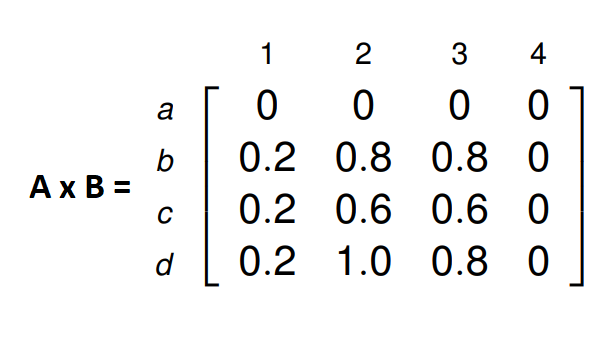And now let us find A' x Y: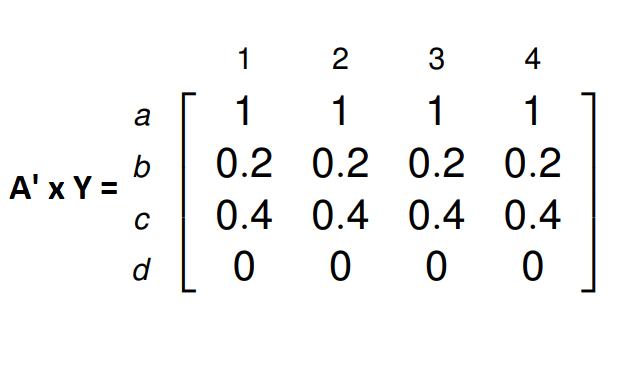Putting these two terms together, we can find R:A → B = (A x B) ∪ (A' x Y) as: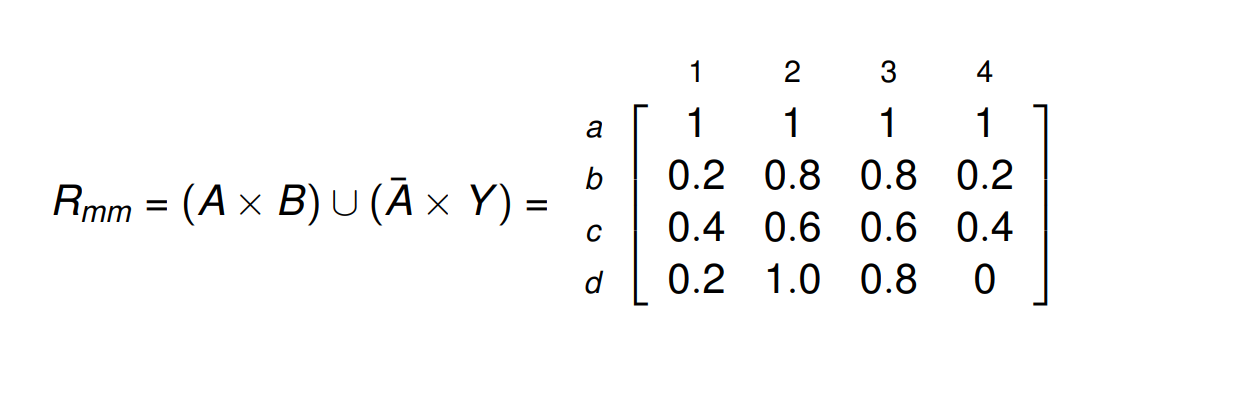Now that we know about fuzzy relations, the different types of operations that can be performed on them, the truth values of fuzzy propositions, and fuzzy implications or if-then rules, we are equipped to learn about how we can draw meaningful inferences from fuzzy systems.

## Fuzzy Inferences

We use two inferring procedures in fuzzy systems:

### 1. Generalized Modus Ponens (GMP)

If we have the statement:
If x is A then y is B.
And if we are given:
x is A'.

Then, by using Generalized Modus Ponens, we can infer:
y is B'.

How can this be done?

We have to find B', which, from Zadeh's Max-Min Rule can be written as:
B' = A' ◦ R(x,y), where R(x,y) = max {(A x B),(A' x Y)} (1)

Let us take a look at an example to understand this better.
Let's consider two fuzzy sets A and B, defined on universes of discourse X and Y respectively. Now, assume that a proposition - 'If x is A then y is B' is given to us, where:
A = {(x1, 0.5),(x2, 1),(x3, 0.6)}
B = {(y1, 1),(y2, 0.4)}
Further, assume that we are given:
x is A', where A' = {(x1, 0.6),(x2, 0.9),(x3, 0.7)}.
From this, we are to derive a conclusion of the form:
y is B', using Generalized Modus Ponens.

From equation (1), we know that we first have to find (A x B) and then (A' x Y), after which we have to perform the composition of A' and the maximum of (A x B) and (A' x Y).
First, let us find (A x B).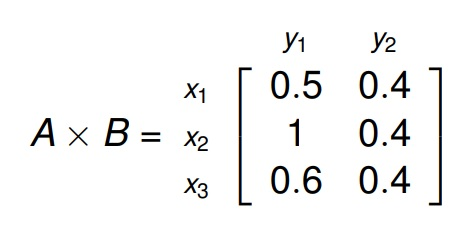Now, let us find (A' x Y).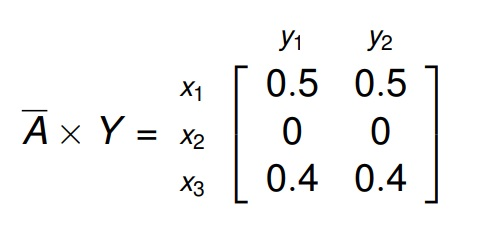Now that we have (A x B) and (A' x Y), we can find R(x,y) = max {(A x B),(A' x Y)}.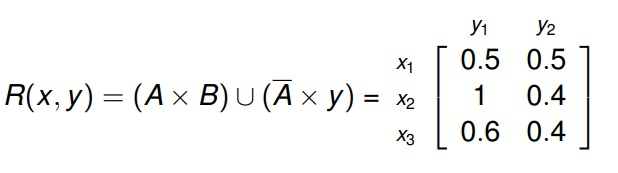And finally, we can perform the composition of A' and R(x,y).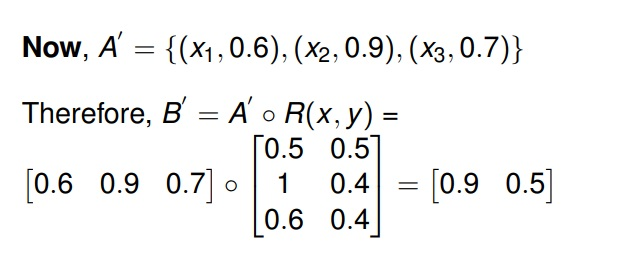Thus, we derive that y is B' where B' = {(y1, 0.9),(y2, 0.5)}.

### Generalized Modus Tollens (GMT)

This inference procedure is very similar to GMP. The only difference lies in the methods of application of these two procedures. Here, if we have the statement:
If x is A then y is B.
And if we are given:
y is B'.

Then, by using Generalized Modus Tollens, we can infer:
x is A'.

Let's consider an example.

Assume that a proposition - 'If x is A then y is B' is given to us, where:
A = {(x1, 0.5),(x2, 1.0),(x3, 0.6)}
B = {(y1, 0.6),(y2, 0.4)}
Now, assume that we are given:
y is B', where B' = {(y1, 0.9),(y2, 0.7)}.
From this information, we are to infer:
x is A', using Generalized Modus Tollens.

Hence, we first calculate R(x,y) = max{(A × B),(A' × y)}.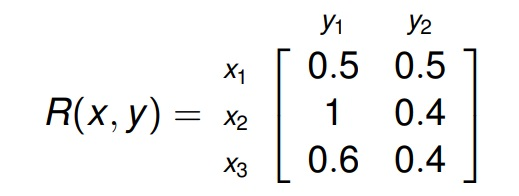Now that we have R(x,y), we can calculate A', which is equal to B' ◦ R(x, y).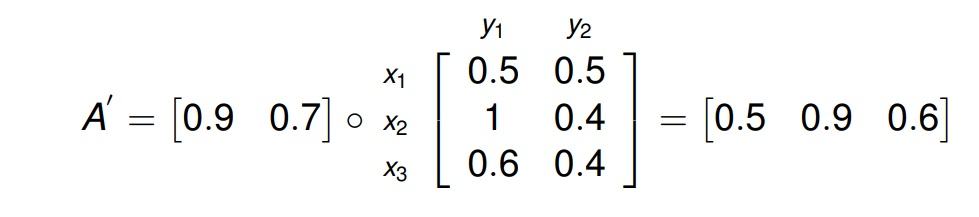Thus, we derive that x is A' where A' = {(x1, 0.5),(x2, 0.9),(x3, 0.6)}.

## Conclusion

In this article at OpenGenus, we learned about fuzzy relations and how we can perform certain operations on them such as the union, intersection and complement operations. We also learned about fuzzy propositions and the truth values of such statements, fuzzy implications, and finally, fuzzy inferences. With this, we now have a clear idea about how we can implement and work with fuzzy systems in the real world.